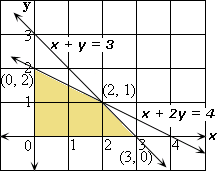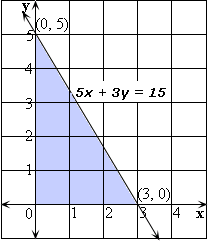## Definition Of Linear Programming

Linear Programming is the process of finding the extreme values (maximum and minimum values) of a function for a region defined by inequalities.

### Example of Linear Programming

Consider the function D = 5x + 4y.
Let us find the minimum value of this function in the region defined by the inequalities
x ≥ 0, y ≥ 0, x + 2y ≤ 4, and x + y ≤ 3.
The feasible region determined by the given constraints is shown.

### Video Examples: Linear ProgrammingThe vertices are (0,0), (3, 0), (2, 1), and (0, 2).
To find the minimum and maximum value of D, we evaluate the value of D = 5x + 4y at each of the four vertices and we find that the minimum value of the function subject to the constraints is 0.
The process that we adopted in the example above is called Linear Programming.

A. 18
B. 8
C. 5
D. 19

### Solution:

Step 1: Objective function is C = 6x + y
Step 2: Constraints are x ≥ 0, y ≥ 0 5x + 3y ≤ 15
Step 3: [Draw the graph.]
The feasible region determined by the given constraints is shown.Step 4: From the graph, the three vertices are (0, 0), (3, 0), and (0, 5).
Step 5: To evaluate the minimum, maximum values of C, we evaluate C = 2x + y at each of the vertices.
Step 6: [Substitute the values.]
At (0, 0) , C = 6(0) + (0) = 0
Step 7: [Substitute the values.]
At (3, 0) , C = 6(3) + (0) = 18
Step 8: [Substitute the values.]
At (0, 5) , C = 6(0) + (5) = 5
Step 9: So, the maximum value of C is 18.